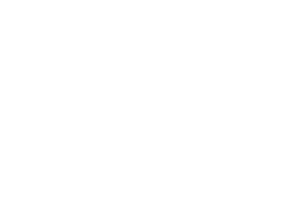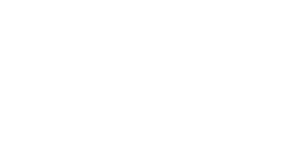Drag

# 计算机图形学代写｜Computer Graphics (COMP3271) Written Assignment 2

1. (20 marks)

(a) Show the difference between perspective projection and orthogonal projection meth
ods by discussing their transformation matrices and rendering results.

(b) Describe the Z-buffer algorithm briefly, and explain how it is used for hidden surface
removal.

2. (20 marks)

(a) Consider a scene with 8 objects (numbered 1 to 8) as shown in the figure below.
Construct the corresponding BSP tree.

(b) Based on the BSP tree, give the back-to-front rendering sequence of the objects from
the viewpoints Eye1 and Eye2, respectively.3. (20 marks)

(a) Describe the three basic components of Phong illumination equation;
(b) Describe how the Flat shading method and Gouraud shading method work;
(c) Describe the pros and cons of Phong shading method.

4. (10 marks)

Consider a texture pattern defined in a unit square [0, 1]2 and a cylindrical surface S, as
depicted in the figure below.The cylindrical coordinates of a point on S can be represented as (r cos ✓, r sin ✓, z), where
r is the radius of the cylindrical surface and. Suppose we want to
wrap the texture on S. Derive a mapping which assigns a texture coorinates in [0, 1]2 to
a point on S.

5. (10 marks) Describe the pros and cons of screen-door transparency compared with
alpha-blending.

6. (20 marks)

(a) Explain the problem produced by using projective shadows on planes, and show how
stencil buffer can help solve the problem;

(b) Show the failure case of shadow volume method, and explain why it fails in this case;

(c) Describe the cons of shadow map method compared with shadow volume and explain
the reason.